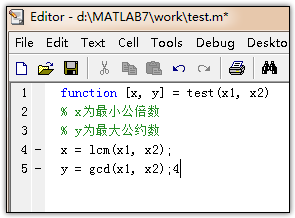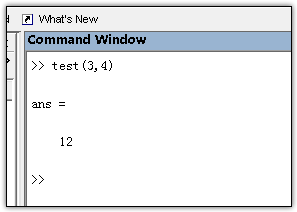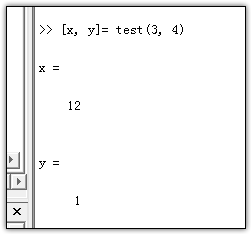• 新建一个.m文件，复制以下代码即可定义函数PlotConfusion，直接按要求调用即可： function F1=PlotConfusion(confusion_matrix) %输入混淆矩阵，绘制混淆矩阵图 %列的方向是真实值，行的方向是预测值 %或者说是x轴为...
新建一个.m文件，复制以下代码即可定义函数PlotConfusion，直接按要求调用即可：
function F1=PlotConfusion(confusion_matrix)
%输入混淆矩阵，绘制混淆矩阵图
%列的方向是真实值，行的方向是预测值
%或者说是x轴为真实标签，y轴是预测标签，原点在左上角
%返回值F1是每个类别的recall和precision的调和平均值，按行排列
%例如：f1=PlotConfusion([12,3;15,2]);

[label_num,~]=size(confusion_matrix);
sum_lable=sum(confusion_matrix);
sum_pre_lable=sum((confusion_matrix'));
precision=zeros(1,label_num);
recall=zeros(1,label_num);
F1=zeros(1,label_num);
%构造plotconfusion函数的输入
labels=[];
predicted_labels=[];
for col=1:label_num
for row=1:label_num
num=confusion_matrix(row,col);
%计算recall和precision
if(row==col)
recall(row)=num/sum_lable(row);
precision(row)=num/sum_pre_lable(row);
end
%构造过程
temp=zeros(label_num,num);
temp_pre=temp;
one_row=ones(1,num);
temp(col,:)=one_row;
temp_pre(row,:)=one_row;
labels=[labels,temp];
predicted_labels=[predicted_labels,temp_pre];
end
end
%绘制混淆矩阵
plotconfusion(labels,predicted_labels);

%计算各类F1指标
for i=1:label_num
F1(i)=2*recall(i)*precision(i)/(recall(i)+precision(i));
end

end



展开全文机器学习
• Matlab提供了强大的函数库供用户调用，但也支持用户自定义函数。本文使用了范德堡大学教授Akos Ledeczi授课中的样例来一步步说明怎样在Matlab中自定义函数。 首先，在command window中输入edit命令。開始编辑...


Matlab提供了强大的函数库供用户调用，但也支持用户自定义函数。本文使用了范德堡大学教授Akos Ledeczi授课中的样例来一步步说明怎样在Matlab中自定义函数。

首先，在command window中输入edit命令。開始编辑函数。本函数实现的是随机生成一个3行4列的矩阵。该矩阵的元素取值范围在0-1之间。

function myRand
a = rand(3,4)
end当中，function和end是keyword，function表示该文件是一个函数，end表示函数体的结束。在command window中运行myRand命令，得到的结果例如以下：

>> myRand
a =
0.5688    0.3371    0.3112    0.6020
0.4694    0.1622    0.5285    0.2630
0.0119    0.7943    0.1656    0.6541
然后，为函数添加參数。本函数实现的是随机生成一个3行4列的矩阵，该矩阵的元素取值范围在low-high之间。

function a = myRand(low, high)
a = low+rand(3,4)*(high - low);
end当中。low和high是函数体的两个输入參数。分别代表了矩阵元素取值范围的上限和下限；a是函数的范围值。在command window中运行myRand命令。得到的结果例如以下：>> myRand(1,6)
ans =
4.4461    1.4191    1.7619    5.9807
4.7408    2.1449    5.1291    1.3909
3.2527    5.5667    3.6917    3.2134

最后，为函数再添加一个返回參数。该函数将随机生成的矩阵中的每个元素相加。返回其总和。

function [a, s] = myRand(low, high)
a = low + rand(3,4)*(high-low);
v = a(:);
s = sum(v);
end当中。s是新添加的返回參数。在command window中运行myRand命令，得到的结果例如以下：

>> [x ss] = myRand(2,4)
x =
3.1504    2.7063    2.0860    3.4634
2.1196    3.6424    2.3380    3.2955
2.4696    2.0308    3.2982    2.9018
ss =
33.5021
到此为止。在Matlab中简单自己定义函数的方法已经介绍完成。Matlab还支持常见的for-loop和if-else等循环和推断结构。參见兴许博客。

转载于:https://www.cnblogs.com/lcchuguo/p/5221815.html
展开全文• matlab支持多输入多输出，函数的返回值可以为一个向量，矩阵，或者多个元素。为了让函数可以调用，需要对函数进行命名。 function [输出变量] = 函数名称(输入变量） 即包含： 输入参数 输出参数 函数名 注释 ...
matlab支持多输入多输出，函数的返回值可以为一个向量，矩阵，或者多个元素。为了让函数可以调用，需要对函数进行命名。
function [输出变量] = 函数名称(输入变量）
即包含：
输入参数
输出参数
函数名
注释
函数体
如图、
回到主窗口，在Command Window中，输入test(3,4)也就是输入函数名称和自变量，就会得到因变量，因变量在函数体中，应该和自变量有个关系式，
因变量=一个自变量的式子
而如果我们输入[x, y] = test(3, 4),则显示如下这说明如果对于多参数输出的函数，如果没有指明接受参数，那么系统只默认接收第一个参数并存至ans变量中。
展开全文• 1. Python调Matlab函数输入matrix 可做如下转换： 假设matlab中某一个函数的输入参数是多维矩阵，那我们可以把python中定义好的数组先转成list，如下： np_a = np.array([[1, 2, 3], [4, 5, 6], [7, 8, 9]]) mat_a =...
1. Python调Matlab函数输入matrix
可做如下转换：
假设matlab中某一个函数的输入参数是多维矩阵，那我们可以把python中定义好的数组先转成list，如下：
np_a = np.array([[1, 2, 3], [4, 5, 6], [7, 8, 9]])
mat_a = matlab.double(np_a.tolist())

这样生成的mat_a就可以直接作为参数传入matlab函数了。
2.Matlab生成的矩阵导入Python
#该方法适用于取得了和matlab的通信接口
h.execute("save('mat_path', 'mat_name');")
q = np.array(mat_path)
mat_a = m['mat_name']

方法2：通过执行matlab编译好的组件，自然也会产生矩阵输出，如matlab.mlarray.double类型的矩阵，可以通过np.array直接转换


展开全文• 下面就总结一下如何在MATLAB定义函数。同时matlab支持多输入多输出，函数的返回值可以为一个向量，矩阵，或者多个元素。为了让函数可以调用，需要对函数进行命名。matlab支持多输入多输出，函...
• ## MATLAB01:基本的数学运算与矩阵运算

万次阅读 多人点赞 2019-11-12 17:22:52
基本的数学运算与矩阵运算MATLAB基本语法变量变量名保留变量不适合做变量...函数使用MATLAB进行矩阵运算定义矩阵向终端输入矩阵使用冒号运算符创建向量定义特殊矩阵矩阵的索引矩阵的操作操作矩阵的运算符操作矩阵的...
• function 输出形参表=函数名（输入形参表） 注释说明部分 函数体语句 注意：当多个形参时，形参之间用逗号分隔，组成形参表。当输出形参多于一个时，应该用方括号括起来，构成一个输出矩阵函数文件名与函数名自己...
• ## Matlab自定义函数

千次阅读 2015-06-30 11:03:57
Matlab提供了强大的函数库供用户调用，但也支持用户自己定义函数。本文使用了范德堡大学教授Akos Ledeczi授课中的例子来一步步说明如何在Matlab中自定义函数。 首先，在command window中输入edit命令，开始编辑...
• 一、矩阵的表示 在MATLAB中创建矩阵有以下规则： a、矩阵元素必须在”[ ]”内； ...最简单的建立矩阵的方法是从键盘直接输入矩阵的元素，输入的方法按照上面的规则。建立向量的时候可以利用冒号...
• y = f(x) y = 1 4 9 16 25 % 这就是你要的数组答：方法和详细的操作步骤如下： 1、第一步，打开matlab输入a = [1 2 3;2 4 5;6 7 8]，创建具有3行3列的矩阵，见下图，转到下面的步骤。 2、第二步，执行完上面的...
• 一、矩阵的表示 在MATLAB中创建矩阵有以下规则： a、矩阵元素必须在”[ ]”内； b、矩阵的同行元素之间用空格（或”...最简单的建立矩阵的方法是从键盘直接输入矩阵的元素，输入的方法按照上面的规则。建立向量的...
• MATLAB矩阵操作大全 一、矩阵的表示 在MATLAB中创建矩阵有以下规则： a、矩阵元素必须在”[ ]”内； b、矩阵的同行元素之间用空格...最简单的建立矩阵的方法是从键盘直接输入矩阵的元素，输入的方法按照上面的规则...
• 一、矩阵的表示 在MATLAB中创建矩阵有以下规则： a、矩阵元素必须在”[ ]”内； ...b、矩阵的同行元素之间用空格（或”,”）隔开；...d、矩阵的元素可以是...最简单的建立矩阵的方法是从键盘直接输入矩阵的元素，输入
• 函数的基本格式 function 输出形参表=函数名(输入形参表） ...在调用函数时，函数输入输出参数称为实际参数，简称实参。 匿名函数基本格式 函数句柄变量=@（匿名函数输入参数） 匿名函数表达式 例如：定义：f
• 基本的数学运算与矩阵运算MATLAB基本语法变量变量名保留变量不适合做变量名变量不应当覆盖内置函数变量类型数字型变量的显示格式MATLAB命令行使用MATLAB进行数字运算使用MATLAB计算数学表达式MATLAB内置的数学函数...
• ## Matlab矩阵常用操作

万次阅读 2017-12-21 11:00:41
一、矩阵的表示 在MATLAB中创建矩阵有以下规则： a、矩阵元素必须在”[ ]”内； ...b、矩阵的同行元素之间用空格（或”,”）隔开；...d、矩阵的元素可以是...最简单的建立矩阵的方法是从键盘直接输入矩阵的元素，输入基本操作
• ## MATLAB矩阵操作大全

千次阅读 2018-06-30 08:51:41
一、矩阵的表示在MATLAB中创建矩阵有以下规则：a...二，矩阵的创建：1、直接输入法最简单的建立矩阵的方法是从键盘直接输入矩阵的元素，输入的方法按照上面的规则。建立向量的时候可以利用冒号表达式，冒号表达式可...
• 本系列博客适合有一点C语言和线性代数基础的人食用！...MATLAB命令行窗口输入 2.Embedding Functions（嵌入函数） 如：(ans指最近的一个ans) 3.变量命名定义和指定值，eg： A=4 cos=‘this string.’;线性代数 数学建模
• % function输出形参表=函数名(输入形参表) % 注释说明部分 % 函数体语句 % 当有多个形参时，形参之间用逗号分隔，组成形参表。 % 当输出形参多于一个时，应该用方括号括起来，构成一个输 % 出矩阵。 % eg: % 1、...
• 最近在使用matlab做代数计算时，通过定义syms 变量和矩阵运算得到了16个代数方程，分别储存在left和right两个4*4矩阵中。每个left(i)=right(i)即为我想要的一个方程。我想用fprint在命令行窗口输出我计算得到的16个...object
• ## matlab矩阵基本操作

千次阅读 2012-06-18 17:17:36
一、矩阵的表示 在MATLAB中创建矩阵有以下规则： a、矩阵元素必须在”[ ]”内； ...b、矩阵的同行元素之间用空格（或”,”）隔开；...d、矩阵的元素可以是...最简单的建立矩阵的方法是从键盘直接输入矩阵的元素，输入存储 pascal matrix...

# matlab定义函数输入矩阵matlab 订阅